# The QUANTLIFE Procedure (Experimental)

### Confidence Interval

Direct computation of the covariance of the parameter estimators involves a complicated density estimation. Instead, the QUANTLIFE procedure computes confidence intervals for the quantile regression parameters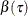by using resampling methods. The QUANTLIFE procedure implements two different methods, the exponentially weighted method and the pairwise resampling method.

#### Exponentially Weighted Method

This method samples weights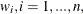from a standard exponential distribution with mean 1 and variance 1. Then it computes the censored quantile regression estimators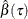based on the observed data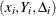with the weights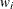. These steps are repeated B times (where B is the value of the NREP= option in the PROC QUANTLIFE statement). The confidence intervals can be obtained from these B estimates. You can specify this method with the CI=EW option in the PROC QUANTLIFE statement.

#### Pairwise Method

This method sampleswith replacement and computes the quantile regression estimatorsbased on the resampled data. These steps are repeated B times (where B is the value of the NREP= option in the PROC QUANTLIFE statement). The confidence intervals can be obtained from these B estimates. You can specify this method with the CI=PW option in the PROC QUANTLIFE statement.

#### Testing Effects of Covariates

Consider the linear model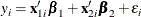where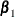and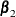are p-dimensional and q-dimensional parameters, respectively, and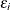,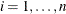, are errors. Denote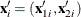, and let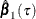and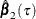be the parameter estimates forand, respectively, at theth quantile.

The QUANTLIFE procedure implements the Wald test for the hypothesis: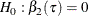The Wald test statistic, which is based on the estimated coefficients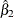from the unrestricted fitted model, is given by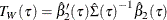where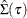is an estimator of the covariance of, which is obtained by using resampling methods.